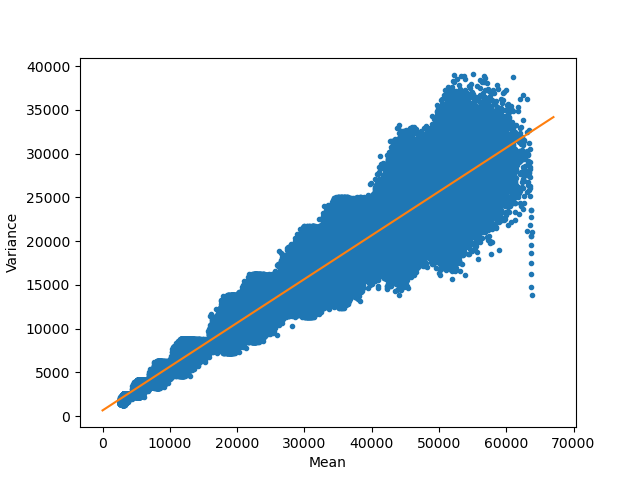In :
%matplotlib notebook
import matplotlib.pyplot as plt
import tifffile
import numpy as np
import os


This notebook shows an example of how to calibrate an EMCCD camera to covert the measured AD converted numbers into number of photons measured. The method is approximate and does not take into account factors such as the quantum efficiency of the camera and readout noise. In summary, the procedure consists of two steps, 1) Determine gain factors to convert signals measured in EM mode to signals measured in normal CCD more 2) Determine conversion factor of measured signal to photons for normal CCD more.

The data can be found online here: https://doi.org/10.5281/zenodo.3765412

In :
data_dir = r'data'

In :
# Function to read all files in a folder and combine them to a stack
def folder_read(path):
return np.stack([tifffile.imread(os.path.join(path, f)) for f in os.listdir(path) if '.tif' in f]).squeeze()


For the first part, we need to relate EMCCD signals to CCD signals. For this we need to illuminate the camera with a constant light source and then tweak the light intensity at the desired EM gain level at low exposure times such that the measured signals are at typical levels for normal measurements. For 16 bit images this would be around 20-40k. Then, while keeping the light intensity fixed, switch to normal CCD mode and adjust exporure time so that the signal is at the level measured before.

The example datasets has measurements at 13 different EM gain levels but only measurments at EM gain levels which you acutally use are required. The same measurement should be done without any light exposure to obtain the dark counts which should be subtracted.

# 1 Determine gain factors¶

To generate lists of the measurement folders:

In :
gain_list = sorted(os.listdir(os.path.join(data_dir, 'gain')), key=lambda x: x.split('_'))
dark_list = sorted(os.listdir(os.path.join(data_dir, 'gain_dark')),  key=lambda x: x.split('_'))
for pair in zip(gain_list, dark_list):
print(pair)

('09_gain100_350ms_1', '01_gain100_350ms_1')
('02_gain1010_45ms_1', '02_gain1010_45ms_1')
('01_gain1200_40ms_1', '01_gain1200_40ms_1')
('08_gain200_190ms_1', '01_gain200_190ms_1')
('12_gain25_1150ms_1', '01_gain25_1150ms_1')
('13_gain30_1000ms_1', '01_gain30_1000ms_1')
('07_gain304_130ms_1', '01_gain304_130ms_1')
('11_gain4_5900ms_1', '13_gain4_5900ms_1')
('06_gain400_110ms_1', '06_gain400_110ms_1')
('10_gain50_650ms_1', '01_gain50_650ms_1')
('05_gain500_90ms_1', '01_gain500_90ms_1')
('04_gain610_75ms_1', '04_gain610_75ms_1')
('03_gain800_60ms_1', '03_gain800_60ms_1')
('14_normalccd_4600ms_1', '14_normalccd_4600ms_1')


The last set of measurements here is the normal CCD which is used to convert to.
To load this dataset:

In :
gain_normal = folder_read(os.path.join(data_dir, 'gain', gain_list[-1]))
dark_normal = folder_read(os.path.join(data_dir, 'gain_dark', dark_list[-1]))
normal = gain_normal.mean(axis=0) - dark_normal.mean(axis=0)
normal.mean(), normal.shape

Out:
(37387.13968906403, (512, 512))

Then we iterate over the other conditions and calculate the gain factor by using the fact that signal is linear with exposure:

$$\frac{Signal_{EM} Exposure_{CCD}}{Signal_{CCD} Exposure_{EM}}$$
In :
for gain_p, dark_p in zip(gain_list, dark_list):
gain = folder_read(os.path.join(data_dir, 'gain', gain_p))
dark = folder_read(os.path.join(data_dir, 'gain_dark', dark_p))
current = gain.mean(axis=0) - dark.mean(axis=0)
expt = int(gain_p.split('_')[:-2])
gain = (current / normal).mean() * (4600 / expt)
print(gain_p, gain)

09_gain100_350ms_1 12.907372445707544
02_gain1010_45ms_1 95.76446423401536
01_gain1200_40ms_1 112.89731560743977
08_gain200_190ms_1 23.306833403419798
12_gain25_1150ms_1 3.9072155556323755
13_gain30_1000ms_1 4.622374013641523
07_gain304_130ms_1 33.4458314412825
11_gain4_5900ms_1 0.7365565027273838
06_gain400_110ms_1 41.99842917155525
10_gain50_650ms_1 7.131435681910387
05_gain500_90ms_1 50.49955678042803
04_gain610_75ms_1 60.43416432076229
03_gain800_60ms_1 77.15986666957193
14_normalccd_4600ms_1 1.0


These numbers can now be used to calculate signals in CCD mode from signals in EM mode. For example, if a signal of 30k was measured with 1200 EM gain, this would have given a signal of 30000/112.89 = 265. Thus we can use these factors to convert to CCD signals.

# 2 Number of photons in CCD mode¶

Next, we want to know how many photons were detected. For this we use the fact that for a Poisson distribution the mean of the population is equal to its mean. We assume that each photon creates an electron in the CCD which means that these electrons, which eventually give the AD signal, are also poissonian. Therefore there should be a linear relation between the variance and mean of the signal measured, and the slope tells us the conversion factor from signal to photoelectrons.

To do so we need to record short movies at different light exposure levels, subtract dark counts and calculate mean and variance for these measurements.

The available folders are:

In :
folders = [f for f in os.listdir(os.path.join(data_dir, 'std_vs_r')) if not 'darkfield' in f]
folders

Out:
['01_1',
'02_1',
'03_1',
'04_1',
'05_1',
'06_1',
'07_1',
'08_1',
'09_1',
'10_1']

Loading the dark counts:

In :
df = tifffile.imread(os.path.join(data_dir, 'std_vs_r', '10_darkfield_1', '10_darkfield_1_MMStack_Pos0.ome.tif'))

In :
df_mean = df.mean(axis=0)
df_mean.mean()

Out:
1679.9514376640323

We then iterate over all folders and calculate for each stack of 200 frames the variance and mean values for every pixel. These values are then appended to two lists which we'll combine later.

In :
var_list = []
mean_list = []
for f in folders:
print(f)
arr = folder_read(os.path.join(data_dir, 'std_vs_r', f)).astype(float)
arr -= df_mean
print(arr.shape)
stds = np.var(arr, axis=0)
means = np.mean(arr, axis=0)

b1 = np.abs(stds - stds.mean()) <3*np.std(stds)  # Remove outliers with love
var_list.append(stds[b1])
mean_list.append(means[b1])

01_1
(200, 512, 512)
02_1
(200, 512, 512)
03_1
(200, 512, 512)
04_1
(200, 512, 512)
05_1
(200, 512, 512)
06_1
(200, 512, 512)
07_1
(200, 512, 512)
08_1
(200, 512, 512)
09_1
(200, 512, 512)
10_1
(200, 512, 512)

In :
all_var = np.concatenate(var_list).flatten()
all_mean = np.concatenate(mean_list).flatten()


To obtain the slope we'll use numpy's polyfit to fit the data to a straight line:

In :
a, b = np.polyfit(all_mean, all_var, 1)

In :
x_plot = np.linspace(0, 1.05*np.max(all_mean), num=200)

plt.figure()
plt.plot(all_mean, all_var, '.')
plt.plot(x_plot, a*x_plot + b)
plt.xlabel('Mean')
plt.ylabel('Variance')Out:
Text(0, 0.5, 'Variance')

The final value for the slope is:

In :
a

Out:
0.5002699721794235

This number means that for a normal CCD (which is what we used to get the graph above) we get 0.5 counts per electron. So when you measure for example 20000 counts this resulted from 40000 photons.

To get the number of photons for the desired EM level you should multiply this number with the gain factor above.

For gain 1200 this number is 112.897. This number will decrease over time due to EM gain ageing as a result of high light exposure. It possible to correct this by adjusting the voltage in the EM gain register. Consult your camera manufacturer on how to do so.

The factor to convert from AD counts to photons is then for gain 1200:

In :
0.5002699721794224*112.897

Out:
56.47897904914025

Divide your measured AD counts by this number to get photons.